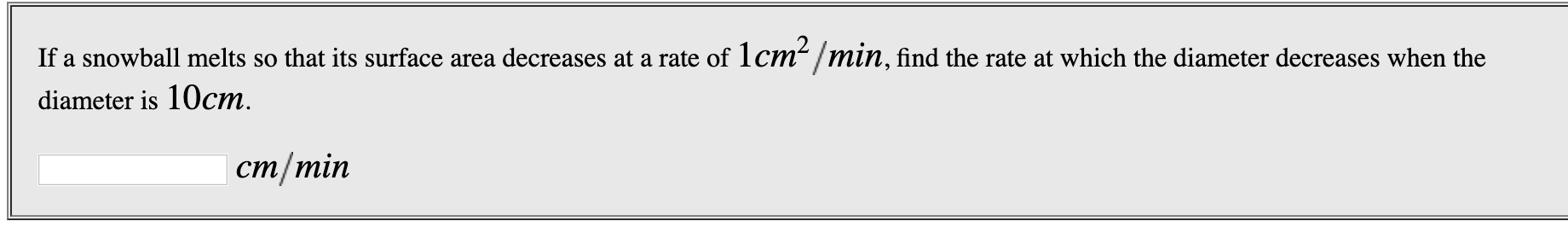# If a snowball melts so that its surface area decreases at a rate of 1cm /min, find the rate at which the diameter decreases when thediameter is 10cmcm/min

Question
11 viewshelp_outlineImage TranscriptioncloseIf a snowball melts so that its surface area decreases at a rate of 1cm /min, find the rate at which the diameter decreases when the diameter is 10cm cm/min fullscreen
check_circle

Step 1

Diameter= D

Surface area = A= 4pi*r^2

Given dA/dt= -1 cm^2/min (negative because decreasing)

Find dD/dt=?

Step 2

Find surface area A in terms of D. ...

### Want to see the full answer?

See Solution

#### Want to see this answer and more?

Solutions are written by subject experts who are available 24/7. Questions are typically answered within 1 hour.*

See Solution
*Response times may vary by subject and question.
Tagged in

### Calculus